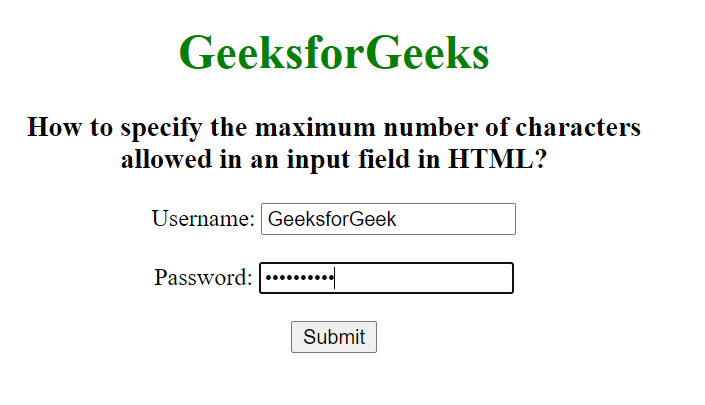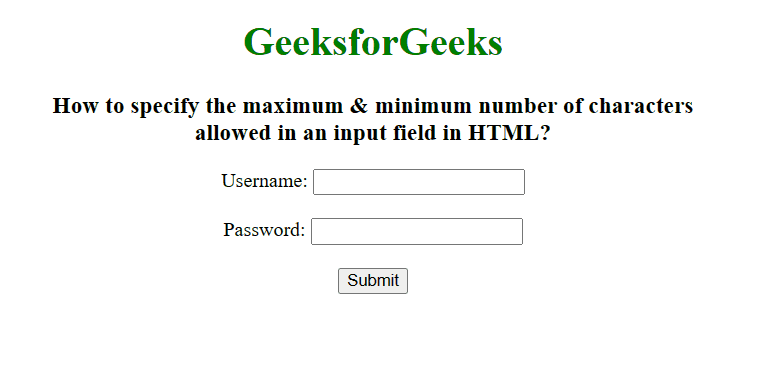GeeksforGeeks App
Open AppBrowser
Continue

# How to specify minimum &maximum number of characters allowed in an input field in HTML ?

In this article, we will learn how to specify the minimum and maximum of characters allowed in an input field in HTML. Given an input field and the task is to set the minimum/maximum number of characters allowed in an input field in HTML.

• To set the maximum character limit in the input field, we use <input> maxlength attribute. This attribute is used to specify the maximum number of characters entered into the <input> element.
• To set the minimum character limit in the input field, we use <input> minlength attribute. This attribute is used to specify the minimum number of characters entered into the <input> element.

Syntax:

```<input maxlength="number1">
<input minlength="number2"> ```

Attribute Value:

• number1: It contains a single value number which allows the maximum number of characters in <input> element. Its default value is 524288.
• number2: The minimum number of characters required in input fields.

Example 1: Set the limit of a maximum number of characters allowed in input fields.

## HTML

 ```<``html``>` `<``head``>``    ``<``style``>``        ``body {``            ``text-align: center;``        ``}``        ``h1 {``            ``color: green;``        ``}``    ``````<``body``>``    ``<``h1``>GeeksforGeeks``    ``<``h3``>``        ``How to specify the maximum number``        ``of characters <``br``>allowed in an``        ``input field in HTML?``    ````    ``<``form` `action``=``"#"``>``        ``Username:``        ``<``input` `type``=``"text"``               ``name``=``"username"``               ``maxlength``=``"12"``>``          ``<``br``><``br``>``        ``Password:``        ``<``input` `type``=``"password"``               ``name``=``"password"``               ``maxlength``=``"10"``>``          ``<``br``><``br``>``        ``<``input` `type``=``"submit"``               ``value``=``"Submit"``>``    ````` ``

Output:Example 2: Set the limit of the maximum & minimum number of characters allowed in input fields.

## HTML

 ```<``html``>``<``head``>``    ``<``style``>``        ``body {``            ``text-align: center;``        ``}``        ``h1 {``            ``color: green;``        ``}``    ``````<``body``>``    ``<``h1``>``          ``GeeksforGeeks``      ````    ``<``h3``>``        ``How to specify the maximum & minimum number``        ``of characters <``br``>allowed in an``        ``input field in HTML?``    ````    ``<``form` `action``=``"#"``>``        ``Username:``        ``<``input` `type``=``"text"``               ``name``=``"username"``               ``minlength``=``"12"``               ``maxlength``=``"20"``><``br``><``br``>``        ``Password:``        ``<``input` `type``=``"password"``               ``name``=``"password"``               ``minlength``=``"10"``               ``maxlength``=``"20"``>``          ``<``br``><``br``>``        ``<``input` `type``=``"submit"` `value``=``"Submit"``>``    ````` ``

Output:My Personal Notes arrow_drop_up
Related Tutorials